# Central Limit Theorem with a Dichotomous Outcome

## Central Limit Theorem with a Dichotomous Outcome

Now suppose we measure a characteristic, X, in a population and that this characteristic is dichotomous (e.g., success of a medical procedure: yes or no) with 30% of the population classified as a success (i.e., p=0.30) as shown below.

The Central Limit Theorem applies even to binomial populations like this provided that the minimum of np and n(1-p) is at least 5, where “n” refers to the sample size, and “p” is the probability of “success” on any given trial. In this case, we will take samples of n=20 with replacement, so min(np, n(1-p)) = min(20(0.3), 20(0.7)) = min(6, 14) = 6. Therefore, the criterion is met.

We saw previously that the population mean and standard deviation for a binomial distribution are:

Mean binomial probability: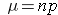Standard deviation: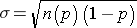The distribution of sample means based on samples of size n=20 is shown below.

The mean of the sample means is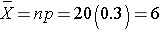and the standard deviation of the sample means is: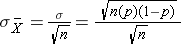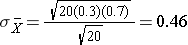Now, instead of taking samples of n=20, suppose we take simple random samples (with replacement) of size n=10. Note that in this scenario we do not meet the sample size requirement for the Central Limit Theorem (i.e., min(np, n(1-p)) = min(10(0.3), 10(0.7)) = min(3, 7) = 3).The distribution of sample means based on samples of size n=10 is shown on the right, and you can see that it is not quite normally distributed. The sample size must be larger in order for the distribution to approach normality.